Chemguide: Core Chemistry 14 - 16 ``` ``` Bond energies ``` ``` This page introduces bond energies and looks at how they can be used to estimate the enthalpy change for some simple reactions. Bond energies are also called bond enthalpies, and in the past have been known as bond strengths. You can take all these terms as meaning the same thing. All the UK syllabuses at this level talk about bond energies, and so I shall stick to this term from now on. If you haven't come across the term "enthalpy change" before, I shall explain that later on this page. ``` ``` Defining bond energies Bond energy is the energy needed to break one mole of a covalent bond to give separated atoms. Everything must be in the gas state. For example, in hydrogen gas there is a single covalent bond between the two hydrogen atoms. To break one mole of that bond to give individual hydrogen atoms with everything in the gas state takes 436 kJ. H2(g)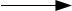2H(g) The bond energy of the H-H bond is 436 kJ mol-1 (kilojoules per mole). The bond enthalpy of the C-H bond varies a bit depending on what compound it is in, and you will find various values within a few kJ of each other from various sources. Don't worry about that. In an exam, use whatever figures your examiners give you. You don't have to remember these values. Ii you break a mole of methane molecules into individual gaseous atoms, it is possible to work out that it would take 1662 kJ. CH4(g)C(g) + 4H(g)    ΔH = +1662 kJ mol-1 That involves breaking 4 C-H bonds per molecule of methane. So the C-H bond strength in methane is 1662/4 = 415.5 kJ mol-1. ``` ``` Breaking bonds is endothermic; making bonds is exothermic This is really important. You have got to get this right, otherwise you won't get bond energy sums right. It is pretty obvious that to break a bond, you will have to put in energy. You can't physically break anything without putting in energy. It isn't so obvious that making bonds releases energy. Let's look at the hydrogen case on an energy diagram.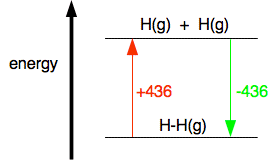Putting in +436 kJ pushes the hydrogen into a higher energy state by breaking the bonds and making individual atoms. Returning to the lower energy state where the atoms are combined is bound to involve the same quantity of energy but now given out again. Anyway: Breaking bonds is an endothermic process. The energy change is positive. Making bonds is an exothermic process. The energy change is negative. Note:  I suggest you learn this on the basis that breaking things needs energy, and making bonds is the opposite of breaking them. ``` ``` Using bond energies to estimate energy changes in reactions Words of warning to start with! You can only use bond energies on their own in reactions which only involve gases. If they involve liquids or solids, you have to use other energy terms as well, and this is beyond the level we are working at. You will occasionally come across an example in a book or elsewhere which does apply bond energies to liquids. That is simply wrong! The other thing that is important is that in most cases the best you can do is to make an estimate of the overall energy change. Bond energies do vary depending on exactly what else is around the bond, and the values quoted in data books are average values which may not exactly apply to the example you are doing. The reaction between hydrogen and fluorine H2(g) + F2(g)2HF(g) The bond energies are: H-H: 436 kJ mol-1 F-F: 158 kJ mol-1 H-F: 562 kJ mol-1 It is usually safer to do these sums by re-drawing the molecules using line diagrams so that you can easily count the bonds. It isn't really necessary in this case, but we will do it anyway.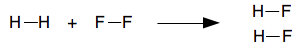Bonds broken 1 H-H: +436 kJ 1 F-F: +158 kJ Bonds made (Remember: making bonds releases heat and so the sign is negative.) 2 x H-F: 2(-562) = -1124 kJ Overall Enthalpy change = +436 + 158 - 1124 = -530 kJ mol-1 A couple of important points, but probably slightly beyond 14-16 level I have introduced the term "enthalpy change" because that is the correct term for what we are estimating. If you have already read the page about measuring the heat evolved or absorbed during a reaction, you will already have come across this. Enthalpy change is simply a measure of the amount of heat evolved or absorbed during a reaction. When you calculate the enthalpy change for a whole reaction, the units are always kJ mol-1. The "per mole" is slightly confusing - "per mole" of what? It refers to the whole equation. If you take the quantities in moles specified by the equation you have written, this is the amount of heat you would expect to be evolved or absorbed. So in this case, if you reacted 1 mole of hydrogen, H2. and 1 mole of fluorine, F2, to get 2 moles of HF, you would get 530 kJ of heat evolved. The negative sign shows that it is evolved. ``` ``` The reaction between methane and chlorine CH4(g) + Cl2(g)CH3Cl(g) + HCl(g) Bond enthalpies: (I am using a data book value for average bond energy for the C-H bond rather than the one I calculated above. That's what you would normally be using.) C-H: 413 kJ mol-1 Cl-Cl: 243 kJ mol-1 C-Cl: 346 kJ mol-1 H-Cl: 432 kJ mol-1 Showing all the bonds: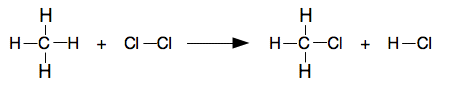Bonds broken 4 C-H: 4(+413) = +1652 kJ 1 Cl-Cl: +243 kJ Bonds made 3 C-H: 3(-413) = -1239 kJ 1 C-Cl: -346 kJ 1 H-Cl: -432 kJ Overall Enthalpy change = +1652 + 243 - 1239 - 346 - 432 = -122 kJ mol-1 Note:  You might have noticed that you have broken 4 C-H bonds and then remade 3 of them. Once you have confidence, you can in fact only think about bonds that are actually changed. Until you are really, really confident, do it the long, safe way by taking everything to pieces and then putting it all together again. ``` ``` Burning propane, C3H8, in air or oxygen C3H8(g) + 5O2(g)3CO2(g) + 4H2O(g) Notice that the equation is showing water as steam rather than liquid water. You can't use bond energies on their own if something is a liquid. I will comment on this again at the end of the sum. This is a good example of where it is really helpful to draw all the bonds. It is easy to get the number of C-C bonds in the propane wrong.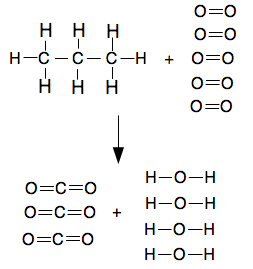Bond enthalpies: C-H: 413 kJ mol-1 C-C: 348 kJ mol-1 O=O: 498 kJ mol-1 C=O (in CO2): 805 kJ mol-1 O-H: 463 kJ mol-1 The calculation Bonds broken 2 C-C: 2(+348) = +696 kJ 8 C-H: 8(+413) = +3304 kJ 5 O=O: 5(+498) = +2490 kJ Bonds made 6 C=O: 6(-805) = -4830 kJ 8 O-H: 8(-463) = -3704 kJ Overall Enthalpy change = +696 + 3304 + 2490 - 4830 - 3704 = -2044 kJ mol-1 A data book gives the enthalpy change of combustion of propane as -2220 kJ mol-1, but that assumes the water is produced as a liquid. When steam condenses to water, 41 kJ mol-1 of heat is given out. Here we have got 4 moles of water, so an extra 4 x 41 = 164 kJ would be evolved. That would make the total enthalpy change -2044 - 164 = -2208 kJ. Why isn't it the same as the data book value? Because bond enthalpy sums are never better than estimates. If you look at the multiplying factors involved in the calculation, a difference of 1 or 2 kJ in a bond energy produces a difference of 8 or 16 in the answer if you multiply it by 8 (as in the O-H value for example). You do not need to know any of this at this level. I am mentioning it out of interest, and to try to stop those of you going on to do chemistry at a higher level having to unlearn badly taught things at this level. ``` ``` Where would you like to go now? To the calculations menu . . . To the Chemistry 14-16 menu . . . To Chemguide Main Menu . . . ``` ``` © Jim Clark 2021